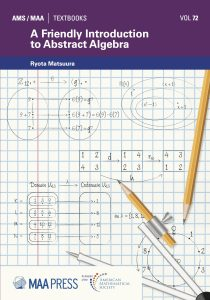# Structure of the Textbook

This textbook is intended to be used in a first-semester course in abstract algebra.Students should have completed Calculus I and II, not because they need the calculus content, but to acquire enough mathematical experience and maturity to handle the abstraction and proof writing that are part of the textbook.

Prior proof-writing experience is not expected. In fact, an underlying goal of the textbook is to guide students toward writing clear and precise mathematical proofs. To support this goal, the textbook includes “Proof know-how,” which are frequent, context-specific, short tips on proof writing. Thus, students learn not only the content, but also the skills and know-how to do mathematics and create new ideas on their own.

Embodying the “experience first” pedagogy, almost all concepts in this textbook are introduced through concrete examples. Ideas are foreshadowed, revisited, and developed over time. For instance, in an exercise about modular arithmetic, students compute the order of units modulo p and make conjectures about these orders. Eight chapters later, the notion of the order of a group element is formally defined. By then, students have seen enough examples so that the concept feels familiar.

The textbook provides students with opportunities to perform experiments and grapple with problems; formulate, test, and revise conjectures; develop theories that bring coherence to observed results; and express understanding using precise mathematical language. In essence, students experience mathematics as a mathematician does.

There are 37 chapters in the textbook. Depending on your students’ background and/or the structure of your algebra course, here are some suggested road maps:

• Chapters 1 through 25 cover group theory. If students have had prior experience with proof writing, you may choose to omit Chapters 1 and 2. Similarly, students may have already studied the notion of divisibility (of integers), particularly if they have taken an introductory number theory course. In such a case, Chapter 3 may be skipped as well. I recommend not skipping Chapter 4 on modular arithmetic, however, since many group-theoretic concepts are introduced in that chapter.
• Chapter 7 contains a brief introduction to matrices and covers matrix concepts that are needed in the textbook. If your students have had a linear algebra course, then Chapter 7 may be omitted or assigned to the students to read on their own as a refresher.
• Chapters 26 through 37 cover ring theory, with an emphasis on polynomial rings. It is possible to end the course with Chapter 35, where we complete the proof of Theorem 35.1 (i.e., F[x]/⟨g(x)⟩ is a field if and only if g(x) is unfactorable).
• Chapters 36 and 37 guide the students to prove Theorem 35.1 again using the notion of maximal ideals. It is a lovely proof, so I recommend its inclusion in your course, if time permits.